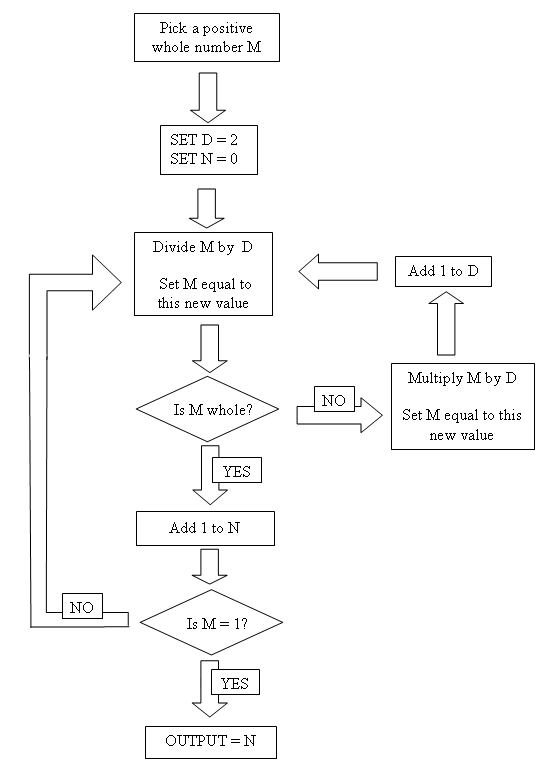#### You may also like### Gaxinta

A number N is divisible by 10, 90, 98 and 882 but it is NOT divisible by 50 or 270 or 686 or 1764. It is also known that N is a factor of 9261000. What is N?### Thirty Six Exactly

The number 12 = 2^2 × 3 has 6 factors. What is the smallest natural number with exactly 36 factors?### Factoring Factorials

Find the highest power of 11 that will divide into 1000! exactly.

# Flow Chart

##### Age 11 to 14 Challenge Level:

Flow charts give very precise instructions, to perform a task or calculation.
The flow chart below uses three variables of which you can choose the starting value for M.

Select several values for M and try to establish what the flow chart does.
A printable version of the flow chart is available here